## Sine-Gordon Equation

A Partial Differential Equation which appears in differential geometry and relativistic field theory. Its name is a pun on its similar form to the Klein-Gordon Equation. The sine-Gordon equation is(1)

whereandare Partial Derivatives. The equation can be transformed by defining(2)(3)

giving(4)

Traveling wave analysis gives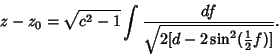(5)

For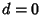,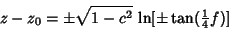(6)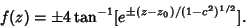(7)

Letting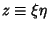then gives(8)

Letting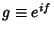gives(9)

which is the third Painlevé Transcendent. Look for a solution of the form(10)

Taking the partial derivatives gives(11)(12)

which can be solved in terms of Elliptic Functions. A single Soliton solution exists with,:(13)

where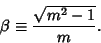(14)

A two-Soliton solution exists with,:(15)

A Soliton-antisoliton solution exists with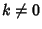,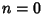,:(16)

A breather'' solution is(17)

References

Infeld, E. and Rowlands, G. Nonlinear Waves, Solitons, and Chaos. Cambridge, England: Cambridge University Press, pp. 199-200, 1990.